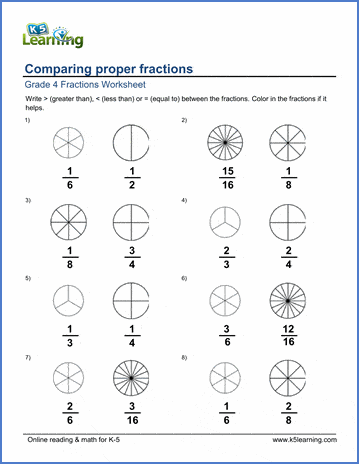# Comparing proper fractions

## Worksheets: Comparing proper fractions (with pie charts)

Below are six versions of our grade 4 fractions worksheet on comparing proper fractions. Students must identify which fraction is larger or whether the 2 fractions are equal.  Fractions have different denominators.  Pie charts are provided and students can color in the charts if needed. Answers are given with the pie charts colored in as an illustration. These math worksheets are pdf files.## More fractions worksheets

Explore all of our fractions worksheets, from dividing shapes into "equal parts" to multiplying and dividing improper fractions and mixed numbers.

## What is K5?

K5 Learning offers reading and math worksheets, workbooks and an online reading and math program for kids in kindergarten to grade 5.  We help your children build good study habits and excel in school.